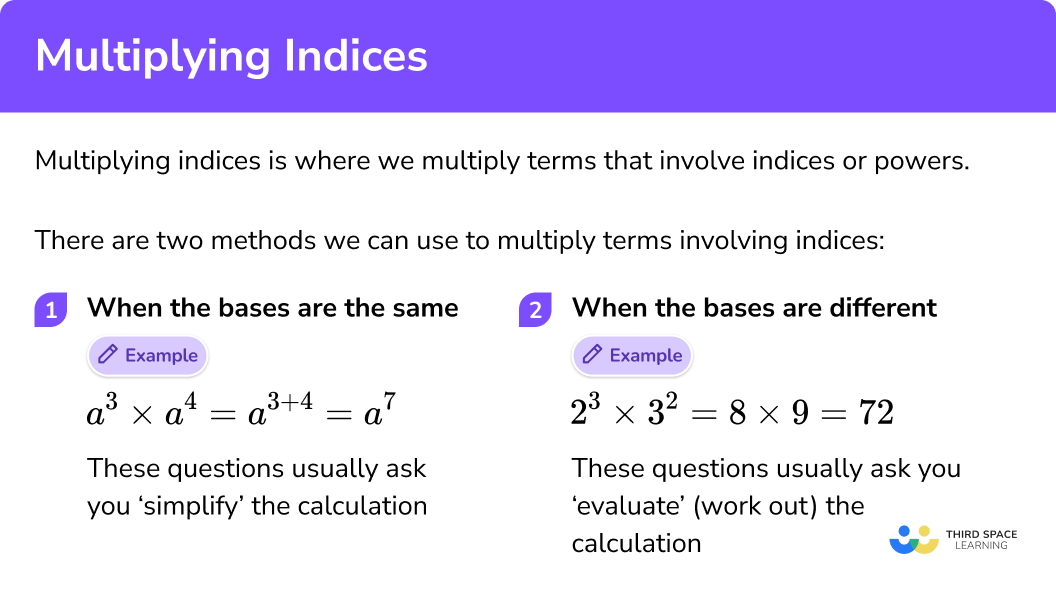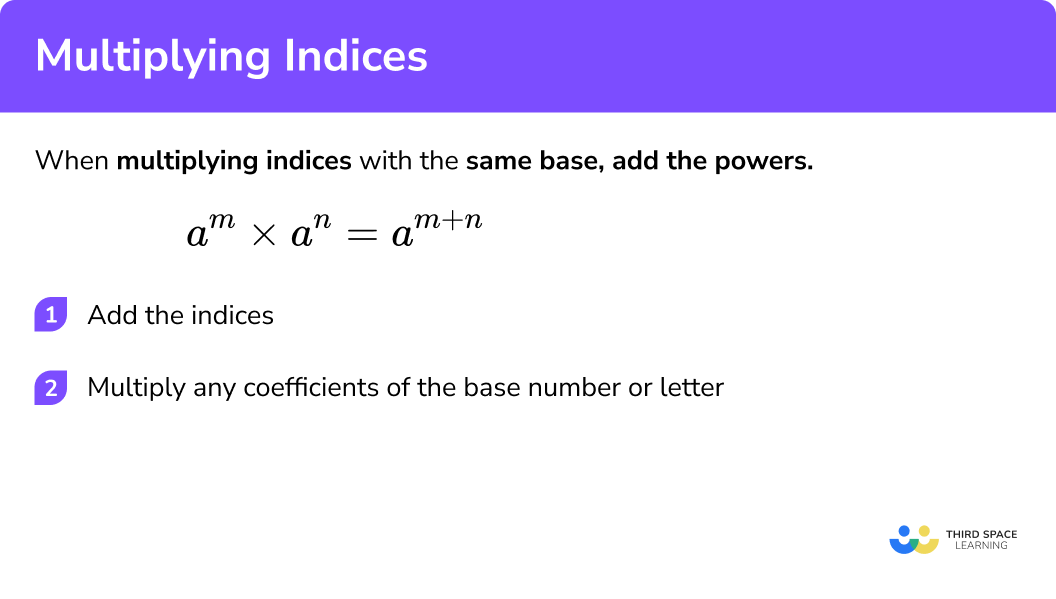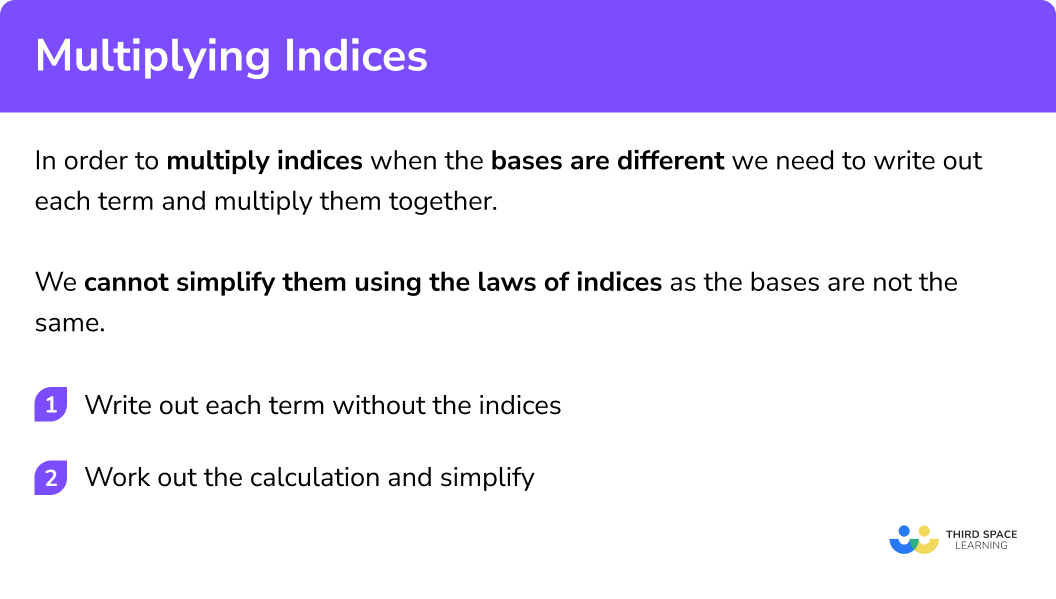GCSE Maths Algebra Laws Of Indices

Multiplying Indices

# Multiplying Indices

Here we will learn everything you need to know about multiplying indices for GCSE maths (Edexcel, AQA and OCR). You’ll learn how to use the laws of indices to multiply indices and how to multiply indices that have different bases.

Look out for the laws of indices worksheets and exam questions at the end.

## What do we mean by multiplying indices?

Multiplying indices is where we multiply terms that involve indices or powers.

There are two methods we can use to multiply terms involving indices.

1. When the bases are the same

E.g.

$a^{3} \times a^{4}=a^{3+4}=a^{7}$

These questions usually ask you ‘simplify’ the calculation

2When the bases are different

E.g.

$2^{3} \times 3^{2}=8 \times 9=72$

These questions usually ask you ‘evaluate’ (work out) the calculation

### What do we mean by multiplying indices?## Related lessons on laws of indices

Multiplying indices is part of our series of lessons to support revision on laws of indices. You may find it helpful to start with the main laws of indices lesson for a summary of what to expect, or use the step by step guides below for further detail on individual topics. Other lessons in this series include:

## How to multiply indices when the bases are the same

In order to multiply indices when the bases are the same we can use the one of the laws of indices.

E.g.

To simplify the following expression:

$8^{3} \times 8^{4}$

We can write out each power in its expanded form.

\begin{aligned} 8^{3} \times 8^{4} &=8 \times 8 \times 8 \times 8 \times 8 \times 8 \times 8 \\ &=8^{7} \end{aligned}

Is there a quicker way to work this out?

Simplifying

$8^{3} \times 8^{4} \text { to } 8^{3+4}$

we can work out the simplified answer is

$8^{7}$

$8^{3} \times 8^{4}=8^{3+4}=8^{7}$

The base has stayed the same and we have added the indices together.

This is the multiplication law of indices.

### How to multiply indices when the bases are the same## Multiplying indices examples (with the same base)

### Example 1: the bases have a coefficient of 1

$a^{5} \times a^{2}$

$5 + 2 = 7$

### Example 2: the bases have a coefficient greater than 1

$4 a^{3} \times 7 a^{2}$

This can be written as

$4 a^{3} \times 7 a^{2}=4 \times a \times a \times a \times 7 \times a \times a$

Add together the indices 3 and the 2.

Multiply 4 and 7 together.

### Example 3: with negative indices

$7 a^{-6} \times 9 a^{-2}$

Add together the indices -6 and the -2.

Multiply the 7 and 9 together.

### Example 4: with algebraic indices

$6 a^{6 b} \times-2 a^{-7 b}$

Add together the indices 6b and -7b.

Multiply the 6 and -2 together.

### Example 5: with an unknown power

This example uses the Bracket Law of Indices. It is a good idea to check our Laws of Indices page for more information before attempting this question.

Step-by-Step: laws of Indices

Work out the exact value of n

$8^{\frac{1}{3}} \times 2^{n}=4^{3}$

Although it appears that the bases are different, we can rewrite each term so that it has a base of 2.

Rewrite each term so that it has the same base.

Use the multiplication law of indices to work out the unknown power.

### Practice multiplying indices questions (with the same base)

x^{5} \times x^{2}

x^{10}x^{7}2x^{7}10xThe base numbers are the same, so we can add the indices.

5+2=7

4 b^{3} \times b^{-7}

4b^{-4}4b^{-21}4b^{4}4b^{-10}The product of the coefficients is 4 , and because we have the same base number we can add the index numbers.

3+(-7)=-4

6 a^{5} \times 4 a^{-3}

10a^{2}24a^{-15}10a^{-15}24a^{2}The product of the coefficients is 24 , and because we have the same base number we can add the index numbers.

5+(-3)=2

-8 a^{7 b} \times 3 a^{3 b}

24a^{10b}-24a^{21b}-5a^{10b}-24a^{10b}The product of the coefficients is -24 , and because we have the same base number we can add the index numbers.

7b+3b=10b

5. Work out the exact value of n

27^{\frac{2}{3}} \times 3^{n}=9^{5}

n=5n=8n=\frac{13}{3}n=\frac{10}{3}Using the rules for indices, we can express the equation using the same base number in each term

3^{2}\times3^{n}=3^{10}

This leaves us with an equation to solve for the indices

2+n=10

## How to multiply indices when the bases are different

In order to multiply indices when the bases are different we need to write out each term and multiply them together.

We cannot simplify them using the laws of indices as the bases are not the same.

1. Write out each term without the indices
2. Work out the calculation

E.g.

To evaluate the following expression:

$2^{3} \times 3^{2}$

We need to write each term of the calculation without using index notation.

$2^{3} \times 3^{2}=2 \times 2 \times 2 \times 3 \times 3$

$2^{3}=2 \times 2 \times 2=8 \\ 3^{2}=3 \times 3=9$

We can the work out the final answer by multiplying these numbers together

\begin{aligned} 2^{3} \times 3^{2} &=8 \times 9 \\ &=72 \end{aligned}

### How to multiply indices when the bases are different## Multiplying indices examples (with different bases)

### Example 1: with positive indices

Evaluate:

$4^{2} \times 2^{4}$

1Write out each term without the indices

$4^{2} \times 2^{4}=4 \times 4 \times 2 \times 2 \times 2 \times 2$

2Work out the calculation.

$4^{2}=4 \times 4=16 \\ 2^{4}=2 \times 2 \times 2 \times 2=16$

So,

\begin{aligned} 4^{2} \times 2^{4} &=16 \times 16 \\ &=256 \end{aligned}

### Example 2: with three terms

Evaluate:

$3^{2} \times 2^{3} \times 10^{2}$

Write out each term without the indices

Work out the calculation.

### Example 3: with negative powers and fractional powers

This example uses the Negative and Fractional Law of Indices. It is a good idea to check our Laws of Indices page for more information before attempting this question.

Evaluate:

$8^{\frac{1}{3}} \times 2^{-2}$

Write out each term without the indices

Work out the calculation.

### Practice multiplying indices questions (with different bases)

1. Evaluate

3^{3} \times 2^{2}

6^{6}6^{5}10836As the base numbers are different, we can evaluate each term and then multiply

3^{3} \times 2^{2}=27 \times 4=108

2. Evaluate

8^{2} \times 4^{-2}

432^{0}32^{-4}1024As the base numbers are different, we can evaluate each term and then multiply

8^{2} \times 4^{-2}=64 \times \frac{1}{16}=4

3. Evaluate

2^{4} \times 5^{2} \times 3^{3}

30^{9}30^{24}10800720As the base numbers are different, we can evaluate each term and then multiply

2^{4} \times 5^{2} \times 3^{3}=16 \times 25 \times 27 = 10800

4. Evaluate

27^{\frac{2}{3}} \times 3^{-2} \times 2^{3}

162^{-4}8972-972As the base numbers are different, we can evaluate each term and then multiply

27^{\frac{2}{3}} \times 3^{-2} \times 2^{3}=9 \times \frac{1}{9} \times 8=8

### Multiplying indices GCSE exam questions

1. Simplify

x^{2} \times x^{3}

(1 mark)

x^{5}

(1)

2. Simplify

6 h^{3} m^{6} \times 4 h^{4} m^{5}

(2 marks)

h^{7} \text { or } m^{11} seen (evidence of adding powers)

(1)

24 h^{7} m^{11}

(1)

3. Simplify

4 x^{-3} y^{5} \times 3 x^{-2} y^{-3}

(2 marks)

x^{-5} \text { or } y^{2} seen (evidence of adding powers)

(1)

12 x^{-5} y^{2}

(1)

### Common misconceptions

• The multiplying indices law can only be used for terms with the same base

E.g. We cannot simplify

$a^{4} \times b^{3}$

as the bases are different.

• Confusing integer and fractional powers

Raising a term to the power of 2 means we square it.

E.g.

$a^{2}=a \times a$

Raising a term to the power of ½ means we find the square root of it.

E.g.

$a^{\frac{1}{2}}=\pm \sqrt{a}$

Raising a term to the power of 3 means we cube it.

E.g.

$a^{3}=a \times a \times a$

Raising a term to the power of ⅓ means we find the cube root of it.

E.g.

$a^{\frac{1}{3}}=\sqrt{a}$

• Indices, powers or exponents

Indices can also be called powers or exponents.

## Learning checklist

You have now learned how to:

• Simplify expressions involving the laws of indices
• Calculate with roots, and with integer and fractional indices

## Still stuck?

Prepare your KS4 students for maths GCSEs success with Third Space Learning. Weekly online one to one GCSE maths revision lessons delivered by expert maths tutors.

Find out more about our GCSE maths tuition programme.